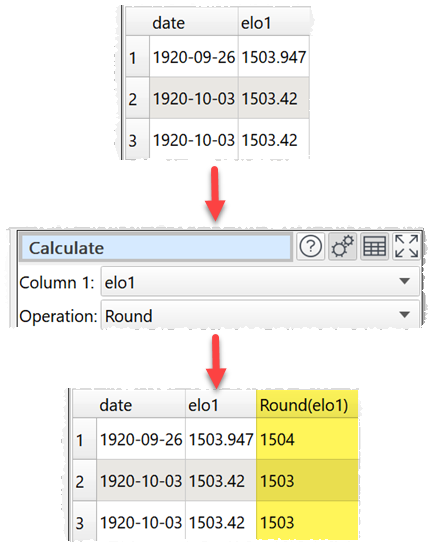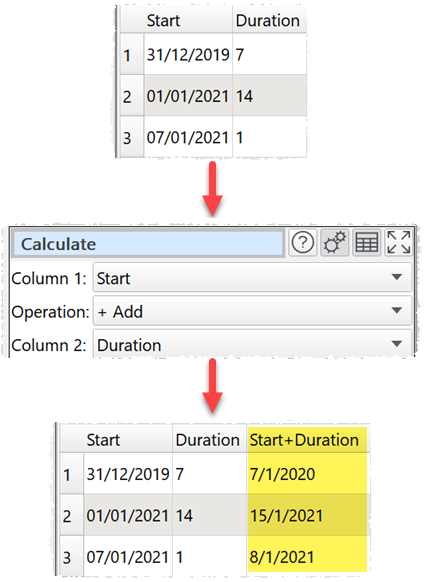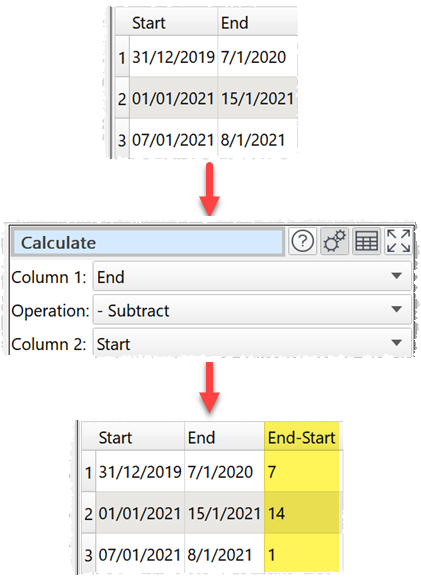﻿ Reference > Transforms > Calculate

# Calculate

## Description

Performs a calculation on 1 or 2 columns and creates a new column.

## Examples

Rounding a column of numbers to the nearest integer:Multiplying 2 columns of numeric values:Adding a days column to a date column:Calculating the difference in days between 2 date columns:One.

## Options

Set Column 1 to the first column you wish to operate on.

Set Operation to the operation you wish to perform.

Set Column 2  the second column you wish to operate on (binary operations only)

## Notes

The following operations are supported.

Operation

Column 1

Column 2

Notes

Examples

Number

Number

1.2 + 3 = 4.2

Date

Integer number

31/01/2021 + 7 = 07/02/2021

- Subtract

Number

Number

Numerical subtraction

1.2 - 3 = -1.8

Date

Integer number

Subtracts days from the date

31/01/2021 - 7 = 24/01/2021

Date

Date

Days the date in Column 1 is after the date in Column 2 (negative if before)

31/01/2021 - 24/01/2021 = 7

24/01/2021 - 31/01/2021 = -7

* Multiply

Number

Number

Numerical multiplication

1.2 * 3 = 3.6

/ Divide

Number

Number

Numerical division

1.2 / 3 = 0.4

1 / 0 = Error

^ Power

Number

Number

Column 1 to the power Column 2 (col1col2)

1.2 ^ 3 = 1.728

2 ^ -3 = 0.125

% Modulus

Integer number

Integer number

Remainder after Column 1 is divided by Column 2

7 % 2 = 1

7 % 0 = Error

Abs

Number

N/A

The absolute value

Abs( 1.2 ) = 1.2

Abs( -1.2 ) = 1.2

Ceiling

Number

N/A

The smallest integer that is not less than the value

Ceiling( 1 ) = 1

Ceiling( 1.2 ) = 2

Ceiling( -1.2 ) = -1

DayOfWeek

Date

N/A

Day of the week, where 1 = Monday and 7 = Sunday

DayOfWeek( 31/01/2021 ) = 7

DayOfWeek( 01/02/2021 ) = 1

DayOfMonth

Date

N/A

Day of the month

DayOfMonth( 31/01/2021 ) = 31

DayOfMonth( 01/02/2021 ) = 1

DayOfYear

Date

N/A

Day of the year

DayOfYear( 01/02/2021 ) = 32

DayOfYear( 31/12/2021 ) = 365

Decrement

Number

N/A

Subtract 1

Decrement( 1 ) = 0

Decrement( -1 ) = -2

Decrement( 1.2 ) = 0.2

Date

N/A

Subtract 1 day

Decrement( 31/01/2021 ) = 30/01/2021

Floor

Number

N/A

The largest integer that is not greater than the value

Floor( 1 ) = 1

Floor( 1.2 ) = 1

Floor( -1.2 ) = -2

Increment

Number

N/A

Increment( 1 ) = 2

Increment( -1 ) = 0

Increment( 1.2 ) = 2.2

Date

N/A

Increment( 31/01/2021 ) = 01/02/2021

Length

Any

N/A

Then number of characters in the value (include any whitespace)

Length( 1.2 ) = 3

Length( 31/01/2021 ) = 10

Length( abcd ) = 4

Ln

Number

N/A

The natural (base e) logarithm of the value

Ln ( 10 ) = 2.302

Ln ( 0 ) = Error

Log10

Number

N/A

The common (base 10) logarithm of the value

Log10 ( 10 ) = 1

Log10 ( 0 ) = Error

Month

Date

N/A

1 = January

Month( 31/12/2021 ) = 12

Round

Number

N/A

Round to the nearest integer

Round( 1 ) = 1

Round( -1.2 ) = -1

Round( 1.5 ) = 2

Sign

Number

N/A

1 if value > 0, 0 if value = 0, -1 of value < 0

Sign( 1.2 ) = 1

Sign( 0 ) = 0

Sign( -1.2 ) = -1

Year

Date

N/A

The 4 digit year

Year( 31/01/2021 ) = 2021

Whether values are interpreted as number, dates or text depends on Supported date formats and Locale in the Preferences window.

To concatenate text in different columns use the Concat Cols transform.

To compare 2 columns use the Compare Cols transform.

For logical operations use the If transform.

For operations on rows or columns use the Stats transform.

For more complex number/date calculations use the JavaScript transform.

To modify the numerical precision of the results use the Num Format transform.

To add a new column of values use the New Col transform.